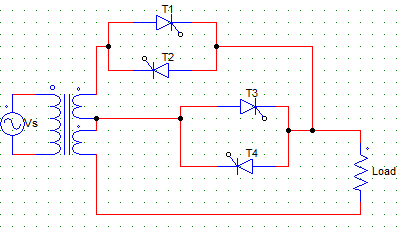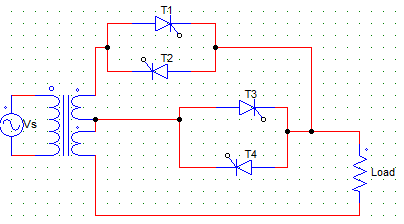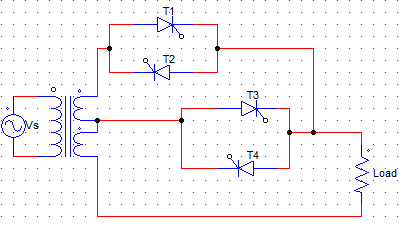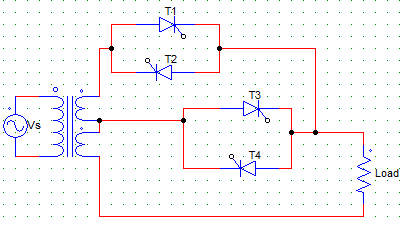Courses

# Test: Sequence Controller

## 10 Questions MCQ Test Power Electronics | Test: Sequence Controller

Description
This mock test of Test: Sequence Controller for Electrical Engineering (EE) helps you for every Electrical Engineering (EE) entrance exam. This contains 10 Multiple Choice Questions for Electrical Engineering (EE) Test: Sequence Controller (mcq) to study with solutions a complete question bank. The solved questions answers in this Test: Sequence Controller quiz give you a good mix of easy questions and tough questions. Electrical Engineering (EE) students definitely take this Test: Sequence Controller exercise for a better result in the exam. You can find other Test: Sequence Controller extra questions, long questions & short questions for Electrical Engineering (EE) on EduRev as well by searching above.
QUESTION: 1

### Sequence control of ac voltage controllers is employed for the improvement of _________

Solution:

It is used to improve the power factor at both the input and output side.

QUESTION: 2

### A two stage sequence control is

Solution:

Sequence control of ac voltage controller means the use of two or more stages of voltage controllers in parallel for the regulation of output voltage.

QUESTION: 3

### From the below given statements regarding sequence control of ac voltage, which of them are true? i) It improves system power factor ii) It reduces the harmonic content at the output iii) Wider control of output voltage is possible

Solution:

All of the above are the advantages of using two or more stages of voltage controllers in parallel. Harmonic content is reduced at two common parameters get cancelled out.

QUESTION: 4

The below given circuit is that of aSolution:

Sequence control of ac voltage controller means the use of two or more stages of voltage controllers in parallel for the regulation of output voltage.

QUESTION: 5

If T1, T2 are kept off and T3, T4 are having a firing angle of 180° each, then the output voltage is ____ if the turns ratio is 1:1Solution:

If T3 and T4 are fired at an angle of 180°, they are equivalent to an off switch, as they are fired and naturally commutated at the same time, hence they are never on.
Hence, as all the four SCRs are always off, the output voltage is zero.

QUESTION: 6

For obtaining the output voltage control from V to 2V, the firing angle must beSolution:

For obtaining the voltage control from V to 2V, the lower controller i.e. T3, T4 pair must always be on hence the firing angle from them must always be 0° ideally.

QUESTION: 7

For obtaining the output voltage control from V to 2V, the firing angle must beSolution:

For obtaining the voltage control from V to 2V, the upper controller must be controlled by varying its firing angle from 0 to 180° whereas the T3, T4 pair must always be on hence the firing angle from them must always be 0° ideally.

QUESTION: 8

A single-phase two stage sequence controller is designed to work on 230 V supply, and upper and lower current ratings must be 20 A and 21 A respectively. Find the transformer rating.

Solution:

Transformer rating is Vs(I1 + I2).

QUESTION: 9

In a N-stage sequence controller, each secondary is rated for __________

Solution:

In a N-stage sequence controller, n voltage controllers are used each having to anti parallel SCR pairs and each secondary is rated for Vs/n.

QUESTION: 10

A single-phase sinusoidal voltage controller has

Solution:

A sinusoidal voltage controller is used to obtain continuous voltage control over wide range with low harmonic content.